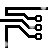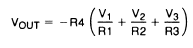## Summing Amplifier

### By internum

The summing amplifier, a special case of the inverting amplifier.

The summing amplifier, a special case of the inverting amplifier, is shown in Figure 1. The circuit gives an inverted output which is equal to the weighted algebraic sum of all three inputs. The gain of any input of this circuit is equal to the ratio of the appropriate input resistor to the feedback resistor, R4. Amplifier bandwidth may be calculated as in the inverting amplifier shown in Figure 1 by assuming the input resistor to be the parallel combination of R1, R2, and R3. Application cautions are the same as for the inverting amplifier. If an uncompensated amplifier is used, compensation is calculated on the basis of this bandwidth as is discussed in the section describing the simple inverting amplifier.Figure 1. Summing AmplifierR5 = R1 || R2 || R3 || R4
For minimum offset error due to input bias current

The advantage of this circuit is that there is no interaction between inputs and operations such as summing and weighted averaging are implemented very easily.139909 December 2007